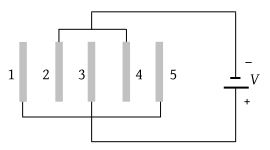The electric potential at a point (x, y) in the xy plane is given by V = –kxy. The field intensity at a distance r from the origin varies as

(1) r2

(2) r

(3) $\frac{1}{r}$

(4) $\frac{1}{{r}^{2}}$

Concept Questions :-

Relation between field and potential
High Yielding Test Series + Question Bank - NEET 2020

Difficulty Level:

Two equal point charges are fixed at x = –a and x = +a on the x-axis. Another point charge Q is placed at the origin. The change in the electrical potential energy of Q, when it is displaced by a small distance x along the x-axis, is approximately proportional to

(1) x

(2) x2

(3) x3

(4) 1/x

Concept Questions :-

Electric potential energy
High Yielding Test Series + Question Bank - NEET 2020

Difficulty Level:

An elementary particle of mass m and charge +e is projected with velocity v at a much more massive particle of charge Ze, where Z > 0. What is the closest possible approach of the incident particle ?

(1) $\frac{Z{e}^{2}}{2\pi {\epsilon }_{0}m{v}^{2}}$

(2) $\frac{Ze}{4\pi {\epsilon }_{0}m{v}^{2}}$

(3) $\frac{Z{e}^{2}}{8\pi {\epsilon }_{0}m{v}^{2}}$

(4) $\frac{Ze}{8\pi {\epsilon }_{0}m{v}^{2}}$

Concept Questions :-

Electric potential energy
High Yielding Test Series + Question Bank - NEET 2020

Difficulty Level:

A solid conducting sphere having a charge Q is surrounded by an uncharged concentric conducting hollow spherical shell. Let the potential difference between the surface of the solid sphere and that of the outer surface of the hollow shell be V. If the shell is now given a charge of –3Q, the new potential difference between the same two surfaces is

(1) V

(2) 2V

(3) 4V

(4) –2V

Concept Questions :-

Electric potential
High Yielding Test Series + Question Bank - NEET 2020

Difficulty Level:

A piece of cloud is having area 25 × 106 m2 and electric potential of 105 volts. If the height of cloud is 0.75 km, then energy of electric field between earth and cloud will be

(1) 250 J

(2) 759 J

(3) 1225 J

(4) 1475 J

Concept Questions :-

Electric potential energy
High Yielding Test Series + Question Bank - NEET 2020

Difficulty Level:

A parallel plate air capacitor has a capacitance of 100 μF. The plates are at a distance d apart. If a slab of thickness $t\left(t\le d\right)$ and dielectric constant 5 is introduced between the parallel plates, then the capacitance will be

(1) 50 μμF

(2) 100 μμF

(3) 200 μμF

(4) 500 μμF

Concept Questions :-

Dielectrics
High Yielding Test Series + Question Bank - NEET 2020

Difficulty Level:

Capacitance of a capacitor made by a thin metal foil is 2 μF. If the foil is folded with paper of thickness 0.15 mm, dielectric constant of paper is 2.5 and width of paper is 400 mm, then length of foil will be

(1) 0.34 m

(2) 1.33 m

(3) 13.4 m

(4) 33.9 m

Concept Questions :-

Dielectrics
High Yielding Test Series + Question Bank - NEET 2020

Difficulty Level:

A parallel plate capacitor is connected to a battery. The plates are pulled apart with a uniform speed. If x is the separation between the plates, the time rate of change of electrostatic energy of the capacitor is proportional to:

(1) x–2

(2) x

(3) x–1

(4) x2

Concept Questions :-

Energy stored in capacitor
High Yielding Test Series + Question Bank - NEET 2020

Difficulty Level:

Five identical plates each of area A are joined as shown in the figure. The distance between the plates is d. The plates are connected to a potential difference of V volts. The charge on plates 1 and 4 will be(1) $\frac{{\epsilon }_{0}AV}{d}.\frac{2{\epsilon }_{0}AV}{d}$

(2) $\frac{{\epsilon }_{0}AV}{d}.\frac{2{\epsilon }_{0}AV}{d}$

(3) $\text{\hspace{0.17em}}\frac{{\epsilon }_{0}AV}{d}.\frac{-2{\epsilon }_{0}AV}{d}$

(4) $\frac{-{\epsilon }_{0}AV}{d}.\frac{-2{\epsilon }_{0}AV}{d}$

Concept Questions :-

Combination of capactiors
High Yielding Test Series + Question Bank - NEET 2020

Difficulty Level:

To form a composite 16 μF, 1000 V capacitor from a supply of identical capacitors marked 8 μF, 250 V, we require a minimum number of capacitors

(1) 40

(2) 32

(3) 8

(4) 2

Concept Questions :-

Combination of capactiors